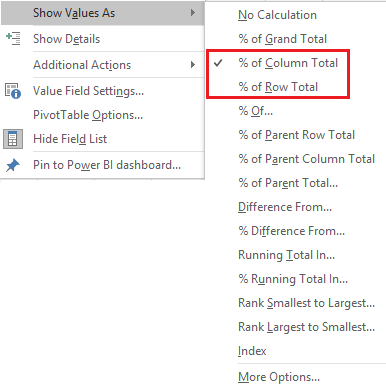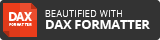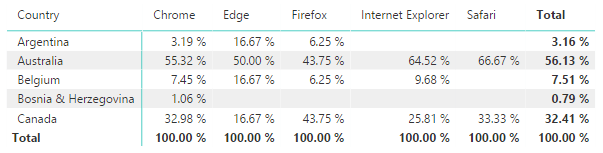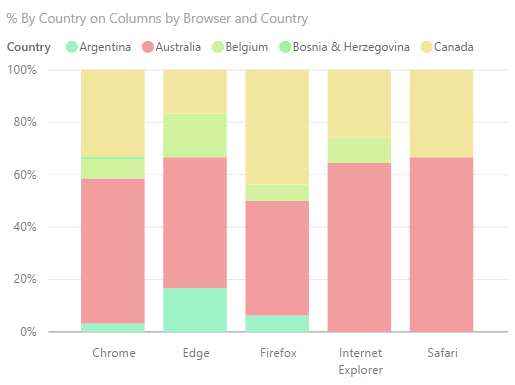Where I am currently consulting there was a requirement to create a measure like you can in the Excel pivot tables for the % of Column Total or the % of Row Total.One of the things that you can currently do in Power BI, which I only learnt as part of this requirement is that you can use % of Column Total, or % of Row Total when using a table, as seen below.But the thing is that as soon as you put that into a visual the only option that you have is the Percent of grand total.

So below are the two measures that I created so that I could then successfully have a % of Column Total (Percent of column total) or % of Row Total (Percent of row total)

# DAX – Percent of Row Total

Below is a picture showing how to get the Percent of Row Total, so that for each row it will add up to 100%Here is the DAX Syntax below, where I did not know that for ALL you could use a table or Column name.

As with the example above I had put the “User Source” on the columns (in a matrix) or Legend (in a Visual)

• DAX Syntax• In the above the [Sessions] is my Measure
• And on the 3rd line is where I am putting in my column that is going to be in the matrix or Legend in the visual.
• NOTE: If I was looking to use this for some other data, I would then replace the [User Source] column with my data I want to add up to 100% on my rows

So that now when this measure is put into a visual it will all add up to 100%# DAX – Percent of Column Total

Below is a picture showing how to get the Percent of Column Total, so that for each column it will add up to 100%Here is the DAX Syntax below, and to know what column I wanted to select, it must be the column which will be on your Rows in your table, or the Axis in a Visual.

As with the example above I had put the “Country” on the columns (in a matrix) or Legend (in a Visual)

• DAX Syntax• In the above the [Sessions] is my Measure
• And on the 3rd line is where I am putting in my column that is going to be in the matrix or Axis in the visual.
• NOTE: If I was looking to use this for some other data, I would then replace the [Country] column with my data I want to add up to 100% on my columns

So that now when this measure is put into a visual it will all add up to 100%. What I did do is to put the Country into the Legend, because this is on Columns for the total to add up to 100%I hope that this is helpful, in that it can enable you or your organization to leverage your data.